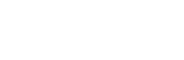#2019-02-16   阅读量: 1894

## 使用xlim后，将“重置为原始视图”缩小到整个范围

xlim在MATLAB中使用时，您设置的限制将成为“原始视图”。

``figure(1); x = 0:0.1:6plot( x, sin(x) ); % Example plot, x axis range [0, 6]xlim( [2, 4] );    % 'Zoom' into the x axis range [2, 4]``

``% Limits are unchanged at [2, 4] when [0, 6] is desirable.``

0.0000 0 2 关注作者 收藏

## 评论(1)2019-02-16

figure(1);

x = 0:0.1:6

plot( x, sin(x) ); % Example plot, x axis range [0, 6]

resetplotview( gca, 'InitializeCurrentView' ) % Ensure we can reset the zoom

xlim( [2, 4] ); % 'Zoom' into the x axis range [2, 4]

0.0000 0 0 回复